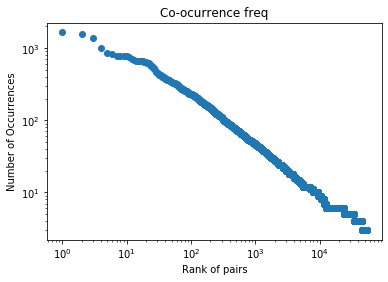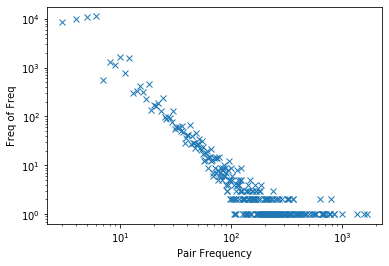# Word Cooccurrence¶

## Cooccurrene_Matrices¶

There are many ways to define distributional word cooccurrence matrices. Here's a schematic overview that highlights the major decisions for building a $word\times word$ matrix:

1. What is a co-occurrence. Cooccurrence happens in a context. The context could be an entire document, a paragraph, a sentence, a clause. In word2vec, it is a sliding window.
2. How to count the co-occurrences. We consider the case that the context is a window of a fixed size $k$. This is the approach that is used by most distributional repreentations. There are several approaches to count the co-occurrences. The simplest method just counts everything in the context window, giving equal weight to every word inside it. A common alternative is to scale the weights based on proximity to the target word – e.g., $(k-d)$, where $d$ is the distance the token from the target, and k is the window size. This method is also used in word2vec. Another method is to using the weight $1/d$. This is used in Glove.

To create the cooccurrence pairs, we scan through the corpus, build a dictionary of word pairs along with their co-occurrence values. Every time a pair of words $w$ and $w′$ occurs in the same context, we incarese the pair weight. It can be 1 (in theory only, never used in prqactice), 1/d, or k-d.

In :
from collections import Counter
import re
import numpy as np
from scipy.sparse import csr_matrix,dok_matrix

In :
def read_vocab(corpus_file, min_count):
vocab = Counter()
with open(corpus_file) as f:
for line in f:
tokens=re.findall('[a-zA-Z]+', line.lower())
vocab.update(Counter(tokens))
return dict([(token, count) for token, count in vocab.items() if count >= min_count])

MIN_COUNT=1
corpus="../data/vldb"
vocab=read_vocab(corpus+".txt", MIN_COUNT)
#vocab

In :
words = list(vocab.keys())
sorted_words = sorted(words)
wi = dict([(w, i) for i, w in enumerate(sorted_words)])
# words and their indexes. For efficiency purpose


### Count cooccurrences within a window¶

Here is an example for counting co-occurrences inside windows. The output is list of (word-pair, freq). Words farther away receive less weight according to (k-d+1), where d is the distance from the center word.

More precisely, for a seqeunce of words

$$w_i w_{i+1}...w_{i+d}$$

The weight of pair (wi, w(i+d)) is k-d+1. I.e., it is proportional to the proximity.

In :
#this one is logically clearer. But need to be improved for large data
def collectPairs(corpus_file, WIN):
counter = Counter()
with open(corpus_file) as f:
for line in f:
tokens=re.findall('[a-zA-Z]+', line.lower())
len_tokens = len(tokens)
for i, tok in enumerate(tokens):
end = i + WIN
if end > len_tokens:
end = len_tokens
for j in range(i+1,end):
distance=j-i
proximity=WIN-distance+1;
if tokens[i] < tokens[j]:
counter[tokens[i]+' '+tokens[j]] +=proximity+1
else:
counter[tokens[j]+' '+tokens[i]] +=proximity+1
return counter

#Pair collection is computationally intensive. We can improve the speed by treating each term as an intger index.
def collectPairs_fast(corpus_file, vocab, wi):
count=0
pair_count = dict()
with open(corpus_file) as f:
for line in f:
tokens=re.findall('[a-zA-Z]+', line.lower())
len_tokens = len(tokens)
i=-1
for tok in tokens:
i+=1
end = i + WIN
if end > len_tokens:
end = len_tokens
w1index=wi[tokens[i]]
for j in range(i+1,end): # range is (i, end) if include the self similarity
w2index=wi[tokens[j]]
v= pair_count.get((w1index, w2index))
val=WIN-(j-i)  # WIN WIN-1, ...,  1
if  v==None:
pair_count[(w1index, w2index)]=val
else:
pair_count[(w1index, w2index)]=v+val
count+=1
if (count%1000000)==0:
print(".", end='',  flush=True)
return pair_count



## Re-Weighting schemes¶

### Construct matrix¶

Once the occurrences are obtained, we can read the counts into a sparse matrix (CSR) from the word-word frequncy count as below.

.. .. wj ..
.. ..
-- -- --- --
wi .. Fij ..
-- -- --- --
.. ..
-- -- --- --

Normally, the raw count $F_{ij}$ are seldom used directly. Often, they are reweighted using metrics such as mutual information. PMI essentially measures the ratio between the observed frequency and expected frequency: $$PMI_{ij} \propto \frac{f_{ij}}{f_if_j}$$

## Efficient implementation¶

dok_matrix is slow when adding elements. It is more efficient to use dict directly, then use update() to transform it into dok_matrix. Note that update() is no longer supported in python3, so we use update() instead. Sppedup is approximately by a factor of 5.

In :
def get_matrix(counter, N, vocab, win,lamb,method):
words = list(vocab.keys())
iw = sorted(words)
wi = dict([(w, i) for i, w in enumerate(iw)])
n=len(wi)
counts = csr_matrix((n, n), dtype=np.float32)
tmp_counts = dok_matrix((n, n), dtype=np.float32)
update_batch_size = 100000
i = 0
factor=N/win/(win-1)/win/(win-1) #

for c in counter:
wa, wb = c.strip().split()
fab = float(counter[c])
fa = vocab[wa]
fb = vocab[wb]
pmi= float(fab)/fa/fb*factor
if method==PMI:
value=pmi
elif (method==SPPMI):
value=pmi/5
else:    #method=DMI
d = lamb/np.sqrt(fab)+1
value= pmi/d

if value>1:  ## keep positive values when they are logged. logging is done in eval.py
tmp_counts[wi[wa], wi[wb]] = value
tmp_counts[wi[wb], wi[wa]] = value
i += 1
if i == update_batch_size:
counts = counts + tmp_counts.tocsr()
tmp_counts = dok_matrix((n, n), dtype=np.float32)
i = 0
counts = counts + tmp_counts.tocsr()
return counts


### Collect word pairs¶

In :
WIN=5
PMI=0
SPPMI=1
DMI=2
METHODS=[PMI, SPPMI, DMI]
mnames=["PMI","SPPMI" , "DMI"]
pairs=collectPairs(corpus+".txt", 5)
n=sum(vocab.values()) #corpus length
N=sum(pairs.values())
N2=n*WIN*(WIN+1)/2 # approximately equal to N when line length is long.
m=len(wi) # voc size

print(N2)
print(N)
print ("Unique pairs:"+str(len(pairs)))

541365.0
476942
Unique pairs:53459

$$w_iw_{i+1}\dots w_{i+k}$$

For each window of of size k, there are $$\sum_{i=1}^k i =\frac{k(k+1)}{2}$$ pairs. If the text is one very long line, the total number of pairs is $$n \frac{k(k+1)}{2}$$

When there are lot of lines, the last a few words in each line will have less pairs. So, the actual pair count should be less than $n k (k+1)/2$.

In :
import matplotlib.pyplot as plt
rank=np.array(range(len(pairs)))+1
pairs_sorted=dict(sorted(pairs.items(), key=lambda item: item, reverse=True))

pair_freq=list(pairs_sorted.values())
plt.loglog(rank, pair_freq, marker='o', linestyle='None')
plt.title("Co-ocurrence freq")
plt.ylabel("Number of Occurrences")
plt.xlabel("Rank of pairs")
plt.show()In :
hist = {}
for i in pair_freq:
hist[i] = hist.get(i, 0) + 1
Y=list(hist.values())
X=list(hist.keys())
plt.loglog(X,Y,marker="x", linestyle='None')
plt.xlabel("Pair Frequency")
plt.ylabel("Freq of Freq")
plt.show()Overall it follows the power law, similar to word frequency distribution. A slight difference can be observed for the rare pairs--The number of pairs that occur only once is similar in size with pairs that occur twice, 3 times and 4 times. For word frequency, hapax is almost twice the count of the words that occur twice.

The reason of less rare pairs is due to the way pairs are taken: pairs only 5 spaces away will have freq 1. Pairs with distances from 1 to 4 automatically have higher counts. Also, there are clusters of five--e.g., pairs with size 5,6,7,8,9 clusters together. They are caused by the way they are counted. Randomized selection as in word2vec will iron out those wrinkles in the plot.

In :
i=0
freq=np.zeros(m)
for w in sorted_words:
freq[i]=vocab[w]
i+=1
pairs=collectPairs_fast(corpus+".txt", vocab, wi)
N=sum(pairs.values())
print(f"{corpus}\tPairs actual={N}\tpairs={N2}\tVoc={len(vocab)}\tcorpus length ={n}\t{N/n}")
factor=2*N/WIN/(WIN-1)/WIN/(WIN-1) # approximate Fi from fi. not accurate when lines are short.

for method in METHODS:
print("\n Creating sparse matrix for "+ mnames[method]+"\t", flush=True)
pairs_val=dict()
i = 0
for w1,w2 in pairs:
Fab =pairs[(w1,w2)]
fa = freq[w1]
fb = freq[w2]
fa_b=fa*fb
pmi= Fab/fa_b*factor
if method==PMI:
value = pmi
elif (method==SPPMI):
value=pmi/5
if value>1:
i+=1
pairs_val[(w1,w2)]=value
pairs_val[(w2,w1)]=value
#if (i%1000000)==0:
#print('.', end='',  flush=True)
tmp_counts = dok_matrix((m, m), dtype=np.float32)
tmp_counts._update(pairs_val)
print("Items:"+ str(i))
matrix = csr_matrix((m, m), dtype=np.float32)
matrix = matrix + tmp_counts.tocsr()
np.savez_compressed(corpus+'_'+mnames[method]+'.mat', data=matrix.data, indices=matrix.indices, indptr=matrix.indptr, shape=matrix.shape)


../data/vldb	Pairs actual=274518	pairs=541365.0	Voc=4583	corpus length =36091	7.606273032057854

Creating sparse matrix for PMI
Items:44405

Creating sparse matrix for SPPMI
Items:28264

Creating sparse matrix for DMI
Items:0


# Project_topics¶

• Co-occurrence counting
• What is the distribution plot of pair counts if pairs are selected using weighting scheme 1/d as in Glove? (you will see that they no longer follow a power law).
• What is the distribution plot if pairs are selected uing a probability as in Word2Vec?
• Can we improve Glove using differnt pair selection methods?
• weighting using arithmetic serials.
• randomized pair selection.
• Matrix reweighting

• Can you coompare the performance for different reweigthing schemes (PMI, SPPMI, etc).
• Shifting. The estimation is not good when pair count is small. One approach is to remove PMI values when the value is small. Can we use the variance as a guideline to remove values that are not certain?

• Disintuish actual observations from multipliers due to the proximity.

# Resources¶

*Omer Levy and Yoav Goldberg. 2014b. Neural word embedding as implicit matrix factorization. In Advances in neural information processing systems, pages 2177–2185.